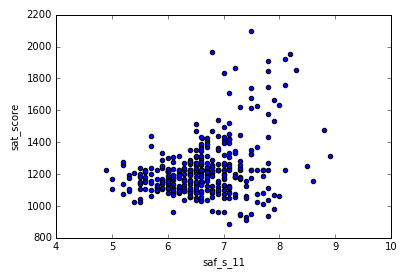# Axes.set_xticklabels and Axes.set_xlabel

Hello,
I’m on the course ‘Exploratory data visualization’. I misunderstood the Difference between Axes.set_xticklabels and Axes.set_xlabel.

`xlabel` refers to the name of the x-axis.

`xticklabel` refers to the interval values along the x-axis!

2 Likes

Thanks!1 Like

Yeah for clarity, here’s with an example from one of the DQ projects:The x label is the `saf_s_11` column name. The xtick labels refer to the intervals occurring at x-axis values of 4, 5, 6, etc.

And welcome to the Dataquest forums!

1 Like

It’s very clear now!1 Like

axes uses the object-oriented api of matplotlib which lets you control the exact axes you want when you have more than 1 axes like when doing subplots with `fig,ax = plt.subplots()`.
If you only have 1 ax, using the state-based `plt` from `import matplotlib.pyplot as plt` directly could be a lot more convenient.
For example https://matplotlib.org/3.1.0/api/_as_gen/matplotlib.pyplot.tick_params.html
`plt.tick_params()` is the analogous higher level api that controls both ticks and ticklabels of the axis (x or y) you specify in the 1st argument. If you use `axes.set_xlabel` to change fontsize you would have to specify the compulsary 1st argument of `labels` while if you did `plt.tick_params('x',fontsize=30)` it will work directly without requiring you to specify labels which could get really long and a pain to type/read. You can understand why if you read the plt.tick_params source code, where it gets the relevant current objects such as tick or ticklabels (depending on what arguments you put inside plt.tick_params) and sets the properties for you.

Another useful tool is `plt.setp()` https://matplotlib.org/3.1.1/api/_as_gen/matplotlib.pyplot.setp.html
where you can set any property on any object. Finding out how to get the object (1st parameter) is the harder part and requires your general understanding of matplotlib anatomy: https://matplotlib.org/3.1.1/tutorials/introductory/usage.html

Just know there are 2 main ways to plot, and google the exact properties they have when you need them. There are still inconsistencies in matplotlib api such as `colors` vs `color` and `rot` vs `rotation` meaning the same thing but requires different spelling when used with different functions

1 Like